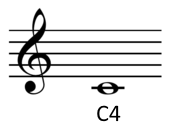# Musical pitch notation

How can you convert the frequency of a sound to musical notation? I wrote in an earlier post how to calculate how many half steps a frequency is above or below middle C, but it would be useful go further have code to output musical pitch notation.

In scientific pitch notation, the C near the threshold of hearing, around 16 Hz, is called C0. The C an octave higher is C1, the next C2, etc. Octaves begin with C; other notes use the octave number of the closest C below.The lowest note on a piano is A0, a major sixth up from C0. Middle C is C4 because it’s 4 octaves above C0. The highest note on a piano is C8.

## Math

A4, the A above middle C, has a frequency of 440 Hz. This is nine half steps above C4, so the pitch of C4 is 440*2-9/12. C0 is four octaves lower, so it’s 2-4 = 1/16 of the pitch of C4. (Details for this calculation and the one below are given in here.)

For a pitch P, the number of half steps from C0 to P is

h = 12 log2(P / C0).

## Software

Here is a page that will let you convert back and forth between frequency and music notation: Music, Hertz, Barks.

If you’d like code rather than just to do one calculation, see the Python code below. It calculates the number of half steps h from C0 up to a pitch, then computes the corresponding pitch notation.

```from math import log2, pow

A4 = 440
C0 = A4*pow(2, -4.75)
name = ["C", "C#", "D", "D#", "E", "F", "F#", "G", "G#", "A", "A#", "B"]

def pitch(freq):
h = round(12*log2(freq/C0))
octave = h // 12
n = h % 12
return name[n] + str(octave)
```

The pitch for A4 is its own variable in case you’d like to modify the code for a different tuning. While 440 is common, it used to be lower in the past, and you’ll sometimes see higher values like 444 today.

If you’d like to port this code to a language that doesn’t have a `log2` function, you can use `log(x)/log(2)` for `log2(x)`.

## Powers of 2

When scientific pitch notation was first introduced, C0 was defined to be exactly 16 Hz, whereas now it works out to around 16.35. The advantage of the original system is that all C’s have frequency a power of 2, i.e. Cn has frequency 2n+4 Hz. The formula above for the number of half steps a pitch is above C0 simplifies to

h = 12 log2P – 48.

If C0 has frequency 16 Hz, the A above middle C has frequency 28.75 = 430.54, a little flat compared to A 440. But using the A 440 standard, C0 = 16 Hz is a convenient and fairly accurate approximation.

## 7 thoughts on “Musical pitch notation”

1. Nice post, John, and very clear. One minor point: in the Baroque period, pitches tended to be low, between A-392 and A-435, but Venetians and others tuned as high as A-465; A-415 is a modern standard for historically informed Baroque performances, but it has little justification — like much “historically informed” performance practice, in my opinion. Many instruments in the nineteenth century were tuned to the Philharmonic pitch, A-452, until A-440 was standardized about the beginning of the 20th century.

2. David Tate

I would hardly call the 37+ cent difference “a little flat” — that’s an ear-grinding miss… And of course this also assumes equal temperament — a topic as fraught with dispute as religion or politics.

Thank you for pointing out that A = 440 is not some kind of natural law. :-)

3. I was going to mention that this assumes universal temperament but it seems others beat me to it. Still, it would be interesting to see comparisons of derivations between different intonation systems (esp between harmonic and universal)

4. There are a lot of complications this doesn’t address: tuning systems, octave stretching, etc. But you can take a frequency like 500 Hz, pass it in, and get B4 out. So that frequency is, approximately, the B above middle C. The exact desired frequency for a B4 is a more complicated matter that depends on context.

5. I was actually looking for more along the lines of what’s in your Circle of Fifths post. Good stuff!

6. Instead of just returning, the nearest pitch, it might be nice to return a tuple containing the note name ad the the number of cents the note is away from the correct pitch.

7. For python 2.x I had to use int function instead of round, as well as the log2 workaround you mentioned